# Fly!

A mini-game where players fly a star among obstacles, provided for you.

BeginnerFull instructions provided1 hour462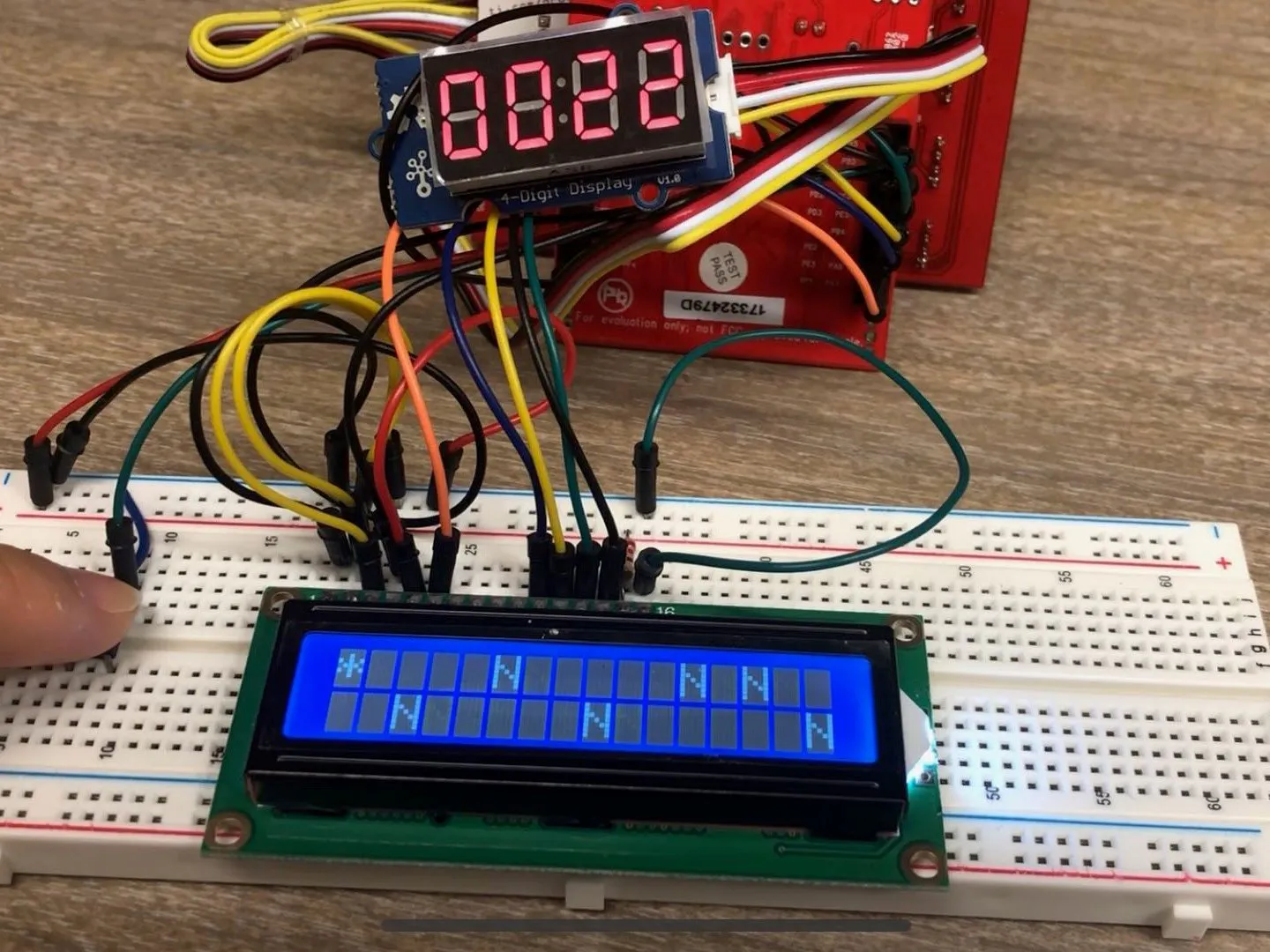## Things used in this project

### Hardware components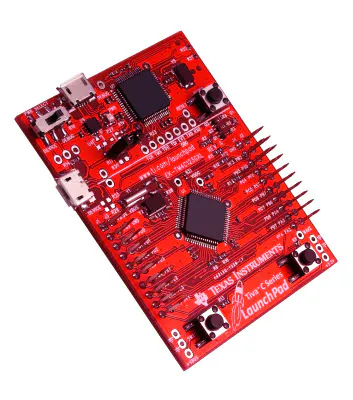Texas Instruments EK-TM4C123GXL TM4C Tiva LaunchPad
×1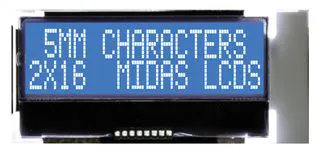Alphanumeric LCD, 16 x 2
×1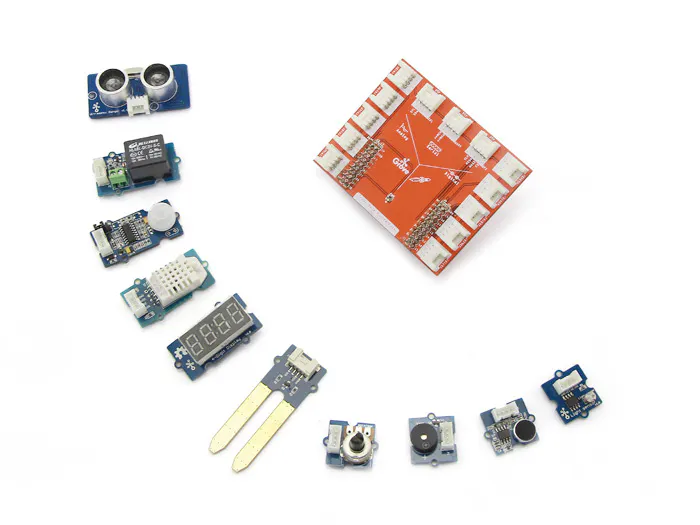Seeed Grove Starter Kit for LaunchPad
×1×1

### Software apps and online servicesTexas Instruments Energia

## Schematics

### Schematic of LCD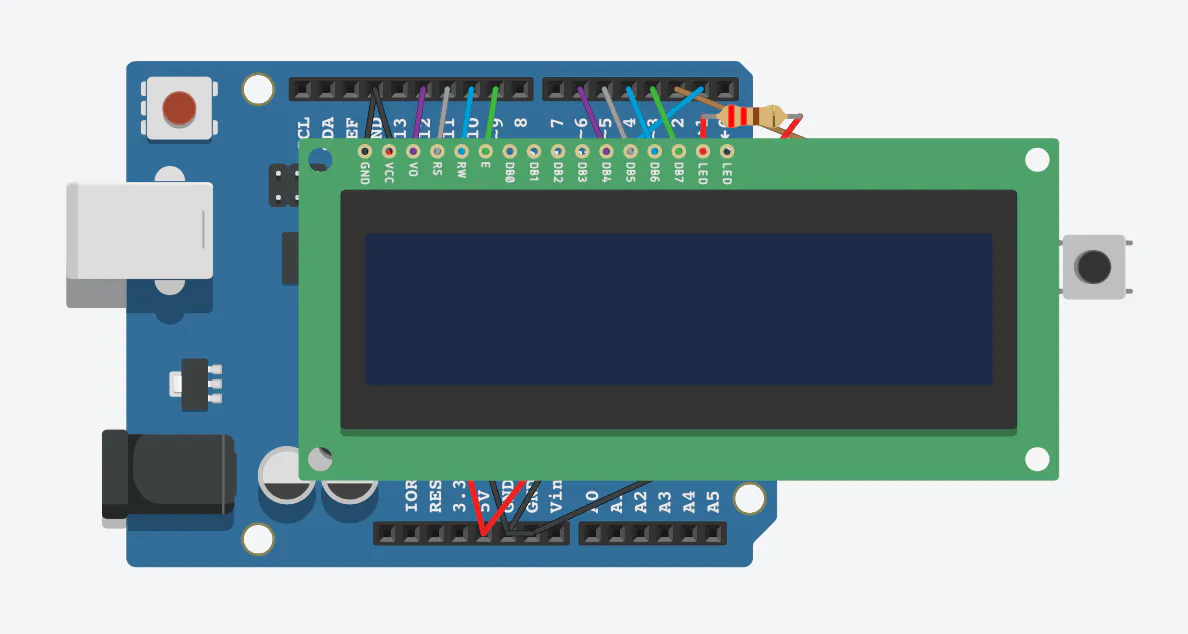## Code

### Full Game Logic

C/C++
200 lines of code do the trick.
```#include <LiquidCrystal.h> //Import the LCD library
#include <stdio.h>

#define LENGTH 16
#define NOBS 10
#define FREQ 200000

#define BUTTONPIN 2             /* pin for the button*/
//LCD thing
LiquidCrystal lcd(11, 9, 6, 5, 4, 3); /*Initialize the LCD and tell it which pins is to be used for communicating*/
#define contra 9 //Define the pin that controls the contrast of the screen
#define bri 3 //Define the pin the controls the brightness of the screen
//Both pins are PWM so you can analogWrite to them and have them output a variable value

//Buzzer thing
#define BUZZER_PIN 38            /* sig pin of the Grove Buzzer */

//Digit display thing
#include "TM1637.h"
#define CLK 36//pins definitions for TM1637 and can be changed to other ports
#define DIO 35
TM1637 tm1637(CLK,DIO);

void setup() {
Serial.begin(9600);
randomSeed(analogRead(2)); // make sure pin 2 not used

//LCD thing
lcd.begin(LENGTH, 2); //Tell the LCD the size
pinMode(contra, OUTPUT); //set pin 9 to OUTPUT
pinMode(bri, OUTPUT); //Set pin 10 to OUTPUT
//pinMode-ing OUTPUT makes the specified pin output power
digitalWrite(contra, LOW); /*outputs no power to the contrast pin.
this lets you see the words*/
analogWrite(bri, 255); //Outputs full power to the screen brightness LED

//Buzzer thing
pinMode(BUZZER_PIN, OUTPUT);

//Button thing
pinMode(BUTTONPIN, INPUT_PULLUP);

//Digit display thing
tm1637.init();
tm1637.set(BRIGHT_TYPICAL);//BRIGHT_TYPICAL = 2,BRIGHT_DARKEST = 0,BRIGHTEST = 7;
}

//Game logic control
void start() {
lcd.setCursor(0,0);
lcd.print(" GAME START! ");
}

void over() {
lcd.clear();
lcd.setCursor(0,0);
lcd.print(" GAME OVER! ");
playGameOver();
lcd.setCursor(0,1);
lcd.print("Restart?");
while (true){
if (buttonState == LOW) {
break;
}
}
}

//Buzzer functions
void playTone(int tone, int duration)
{
for (long i = 0; i < duration; i += tone * 2)
{
digitalWrite(BUZZER_PIN, HIGH);
delayMicroseconds(tone);
digitalWrite(BUZZER_PIN, LOW);
delayMicroseconds(tone);
}
}

void playGameOver(){
playTone(1800,100000);
}

void loop() {
//Round initialization
start();
int score = 0;
int counter = 0;
int buttoncounter = 31;
bool ongoing = true;
int xl[NOBS + 1];
int yl[NOBS + 1];
xl = 0;
yl = 1;
//Generate initial obstacles
for (int i = 1; i < NOBS + 1; i++) {
bool noSolution = true;
int x;
int y;
while (noSolution) {
x = random(LENGTH,LENGTH * 2);
y = random(0,2);
noSolution = false;
for (int j = 1; j <= i; j++) {
if ((yl[j] != y) && (xl[j] == x || xl[j] - 1 == x || xl[j] + 1 == x)) {
noSolution = true;
}
if (xl[j] == x && yl[j] == y) {
noSolution = true;
}
}
}
xl[i] = x;
yl[i] = y;

}
while (ongoing) {
//button
if (buttonState == LOW && buttoncounter > (FREQ / 4)) {
yl = 1 - yl;
//playTone(1700,10);
buttoncounter = 0;
//display
lcd.setCursor(xl,1- yl);
lcd.print(" ");
lcd.setCursor(xl,yl);
lcd.print("*");
playTone(1700,10);
}
//shift obstacles left
if (FREQ - (score * (FREQ / 200)) == counter) {
counter = 0;
for (int i = 1; i < NOBS + 1; i++) {
xl[i]--;
//Update obstacles
if (xl[i] < 0) {
score++;
if (score == 10000) {
score = 0;
}
bool noSolution = true;
int x;
int y;
while (noSolution) {
x = random(LENGTH,LENGTH * 2);
y = random(0,2);
noSolution = false;
for (int j = 1; j < NOBS + 1; j++) {
if ((yl[j] != y) && (xl[j] == x || xl[j] - 1 == x || xl[j] - 2 == x || xl[j] + 1 == x )) {
noSolution = true;
break;
}
if (xl[j] == x && yl[j] == y) {
noSolution = true;
break;
}
}
}
xl[i] = x;
yl[i] = y;
}
}
//display objects
lcd.clear();
lcd.setCursor(xl,yl);
lcd.print("*");
for (int i = 1; i < NOBS + 1; i++) {
lcd.setCursor(xl[i],yl[i]);
lcd.print("N");
}
int8_t segScore;
segScore = score / 1000;
segScore = (score % 1000) / 100;
segScore = (score  % 100) / 10;
segScore = score % 10;
tm1637.display(segScore);
}

//game over control
for (int i = 1; i < NOBS + 1; i++) {
if (xl == xl[i] && yl == yl[i]) {
lcd.setCursor(0,yl);
lcd.print("X");
delay(1000);
ongoing = false;
}
}
counter++;
buttoncounter++;
}
over();
}
```

## Credits

### Maki Yu

2 projects • 1 follower
EECS+Studio Art Student at Rice University

### Wilson Wang

1 project • 0 followers

### Zoey Ling

1 project • 0 followers

### Zhanyi Sun

1 project • 0 followers